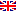Scheda programma d'esame
FUNDAMENTALS OF SIGNALS, SYSTEMS AND NETWORKS
GIANCARLO PRATI
CdSINFORMATICA E NETWORKING
Codice580II
CFU12
PeriodoAnnuale
LinguaInglese

ModuliSettore/iTipoOreDocente/i
FONDAMENTI DI SEGNALI, SISTEMI E RETIING-INF/03LEZIONI100
 GIANCARLO PRATI unimap LUCA VALCARENGHI unimapEsporta in pdf
Obiettivi di apprendimento
Learning outcomes
Conoscenze

This  course  introduces  the  fundamentals  of  signal  theory,  of  stochastic  processes,  the  fundamentals  of queueing theory, some basic elements of electromagnetism and some calculus. The course will also cover the main network architectures for access, metropolitan and core segments.

Knowledge

This  course  introduces  the  fundamentals  of  signal  theory,  of  stochastic  processes,  the  fundamentals  of queueing theory, some basic elements of electromagnetism and some calculus. The course will also cover the main network architectures for access, metropolitan and core segments.

Indicazioni metodologiche

Lectures

Teaching methods

Lectures

Programma (contenuti dell'insegnamento)
• Signal Theory, basic calculus
1. Finite energy and finite power discrete and continuous signals
2. Periodic signals
3. Time invariant linear systems
4. Description of signals and systems in the frequency domain
• Stochastic processes and queueuing theory
1. General concepts
2. Probability and random variables
3. Stochastic processes
4. Markov chain and process
5. Elements of queuing theory
• Design of networks
1. Network hierarchy
2. Access segment
3. Metropolitan segment
4. Core segment
5. Future architectures
• Network hierarchy
• Access segment
1. Introduction and challenges
3. Active optical networks
4. TDM/WDM passive optical networks
• Metro segment
1. Introduction and legacy technologies
2. Emerging technologies (PoS, Carrier Ethernet)
3. WDM metro networks
• Core segment
1. Introduction and optical transport technologies
2. Wavelength switched optical networks
3. WDM network design
4. Optical network control plane: GMPLS
5. Photonics in switching: optical burst/packet switching
• Summary and outlook
Syllabus
• Signal Theory, basic calculus
1. Finite energy and finite power discrete and continuous signals
2. Periodic signals
3. Time invariant linear systems
4. Description of signals and systems in the frequency domain
• Stochastic processes and queueuing theory
1. General concepts
2. Probability and random variables
3. Stochastic processes
4. Markov chain and process
5. Elements of queuing theory
• Design of networks
1. Network hierarchy
2. Access segment
3. Metropolitan segment
4. Core segment
5. Future architectures
• Network hierarchy
• Access segment
1. Introduction and challenges
3. Active optical networks
4. TDM/WDM passive optical networks
• Metro segment
1. Introduction and legacy technologies
2. Emerging technologies (PoS, Carrier Ethernet)
3. WDM metro networks
• Core segment
1. Introduction and optical transport technologies
2. Wavelength switched optical networks
3. WDM network design
4. Optical network control plane: GMPLS
5. Photonics in switching: optical burst/packet switching
• Summary and outlook
Bibliografia e materiale didattico

G. Prati and L. Prati, "Introduction to Stochastic Processes and Queuing Theory", Edizioni ETS, 2014, ISBN 978-884674059-5

Slides from the teacher.

Bibliography

G. Prati and L. Prati, "Introduction to Stochastic Processes and Queuing Theory", Edizioni ETS, 2014, ISBN 978-884674059-5

Slides from the teacher.

Modalità d'esame

Exam consists of a written test concerning course concepts and a possible discussion of a project assigned to  the student.

Assessment methods

Exam consists of a written test concerning course concepts and a possible discussion of a project assigned to  the student.

Ultimo aggiornamento 28/07/2017 10:35